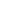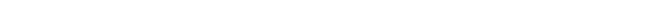## The electron

When you are interested in physics you must read “Unbelievable“!

The pictured process of oscillation and the assumed physical entities of ether are an “unscientific” account of what might happen should ether exist. The sketched image was only necessary to explain physics when there is ether. The images are necessary to understand the meaning of derived mathematical solutions. The math to come must be consistent with observations. The story has the purpose to comprehend the accompanying math.

The best known and smallest elementary particle, apart from the neutrino, is the electron. An electron can have a positive or a negative charge Qe. The electron with a positive charge is called positron. When we mention the electron we mean the negative charged particle.

When we assume a completely separated point-volume in a negative and a positive point-volume this separation is temporarily. The charges will be annihilated the surrounding ether with the speed of light.

To be able to describe and explain a stable positive and negative particle like the electron we have to ascribe to the separated volume energy in the form of rotation energy. Point-volumes can vibrate, but it is also possible that the point-volume rotates around an axis. When a point-volume spins, the rotation represents pure magnetic energy: movement of charge or volume.

In the ether theory it is more consistent to call negative and positive electric charges negative and positive volumes. It is not necessary to introduce an electric charge. It is only a name. We will however conform to the custom and call the separated volumes electric charges.

Imagine now that the oscillation energy of a spinning point-volume is large enough to separate a neutral point-volume completely in a spinning negative and positive point-volume. We consider the spinning negative point-volume, with charge –Qe, further.

The interaction with the ether as described previously will be as follows. The spinning negative point-volume misses positive charge to be neutral. This positive charge is transferred to the point-volume the surrounding point-volumes respectively ether. When the positive charge enters the spinning negative point-volume this absorbed charge will not only neutralize the negative charge, but the absorbed charge will be drawn into a spin together with the spinning negative charge of the point-volume (magnetic induction).

By absorbing the positive charge from surrounding point-volumes the negative point-volume will become neutral again. The negative charge is passed on to the surrounding point-volumes when their positive charge is transmitted. These now partly negative charged point-volumes will attract the charge they transmitted from neutral point-volumes surrounding them, and so on. The negative charge of the original negative point-volume is spread over space with the speed of light. The ether, transferred to the spinning volume, is dragged into the spin. The speed of rotation will be the highest on the outside, of the still negatively charged, spinning and expanding bulb.

Figure 14 presents the profile of the expanding and rotating negative point-volume. The black spot in the center represents the original negative point-volume and the gray area the surface where the negative charge is neutralized and dragged. While expanding, the transferred charge is dragged into the spin.Figure 14.  The spinning and expanding point-volume.

When the rotation energy of the expanding and rotating negative-point-volume is large enough to drag the rotation speed to the velocity c at the end of the profile, the spinning charge at the end of the profile will lose contact with the surrounding ether.

When the rotation speed reaches c the charge at the end of the profile rotates so fast that the inert quality of the ether (c) prevents charge to be dragged any longer. There will still be charge separation at the outside the profile, but the ether cannot pass charge anymore because the transferred charge now has to be accelerated to c.

The inert quality of ether, the lag to transfer charge from one point-volume to another with a speed larger then c makes this impossible. The expansion of the negative charge in space has come to an end. An extremely stable elementary particle, the electron, is born.

It is not possible to explain the Relativity Theory that speed c is reached. The speed of light can only be approached. With ether there is however no objection at all to reach the speed of light for the ether itself, because the speed of light c is one of the characteristics of vacuum/ether. The consequence when the speed of light is reached is that the ether moving with speed of light cannot drag the opposite charged ether anymore. The transfer of charge stops. The phenomenon, the Lorentzfactor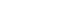, shows its presence again. The ether is too inert to interact in that way with the spinning charge.

The following situation has occurred: surrounding the, now neutralized, negative point-volume in the center of the profile, the dielectric replaced charges turn within the radius Re of the electron. The dielectric displacement at Re has been dragged to the speed of light c.

The ether, from the original point-volume until Re, is in balance. The ether of the spinning electron is comparable with a spinning disc. There is now a situation where the spinning charge on the bulb determines its own space. Within the radius Re of the electron the ether is at rest. The electron is in control of the space, ether, with radius Re. The negative charge –Qe is annihilated inside the sphere with radius Re. The charge -Qe is now positioned at the surface of the bulb with radius Re.Figure 16.  The electron

The induced magnetic spin energy in the ether, surrounding the electron, is directly linked to the electron means of the electrostatic field outside the spinning radius of the electron.

The charge –Qe seeks a low as possible energy level. Hence the electrostatic charge –Qe, situated at the bulb with radius Re, tries to expand. The expanding electrostatic force of the –Qe charged bulb with radius Re is compensated the contracting force of the magnetic spin energy.

Further expansion of the electron above Re is not possible, because than the spin energy in the ether has to diminish (equitation (e1) below). The expanding electrostatic force and the contracting magnetic spin are in equilibrium when the radius of the electron is Re.

The positron emerges in a similar way. The only difference is that the original point-volume is positively charged. The magnetic energy of the spinning disc is given the formula: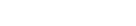(e1)

(For derivation of this formula see below “The Electron Mathematically”)

Where Wm is the magnetic energy of the spinning disc, Qe the charge of an electron, c the speed of light and Re the radius of the electron. If the negative point-volume has not enough energy to drag the ether to the speed of light then a stable situation cannot occur.

The radius Re of the electron does not tell us anything about the size of the original point-volume. Re presents the area of influence of the electron in the ether. Charge –Qe stopped expanding when the dielectric displacement at Re has a pace equal to the speed of light c. The transfer of charge stopped and the negative charge –Qe is now situated on a bulb with radius Re.

The presentation of the electron mathematically is not yet complete. The negative point-volume expanded to Re in all directions with speed c. The electron is created when the velocity is c at Re. The charge –Qe is now situated at a bulb with radius Re. The electrostatic energy Wp of charge –Qe situated on the surface of a bulb with radius Re is in physics is given the formula: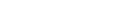The formula of the electrostatic energy Wp is exactly the same as the formula for the magnetic spin energy Wm (equation (e1)), when 1/εo is replaced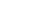according to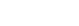.

So the magnetic and the electrostatic energy of the electron are equal. This is not a coincidence because in “nature” energy is divided proportionally equally over the degrees of freedom to store energy. The equipartition principle divides the energy equally over the different forms of energy. The electron can store energy in two ways: electrostatic and magnetic.

The total energy of the electron We is the sum of magnetic and electrostatic energy:

We=Wm+Wp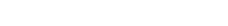Because the first part of this equation is equal to the second part the intrinsic energy of the electron We is equal to: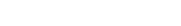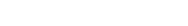and is therefore in accordance with the principle of equivalence of energy and mass.

When the radius Re is calculated from the formula for We we derive: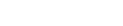meter, exact the classical radius of the electron: the Compton Radius.

The stability of the electron can be deducted from the formula for the total energy of the electron We. When Re increases the energy of the electron becomes less than the energy of the particle and that is not possible; energy cannot be lost.

The physical interpretation is that the induced magnetic energy of the spin captures the charge to the surface of a bulb with radius Re. Outside the electron radius the ether is spinning with the energy of the induced current Wm. The charged bulb with radius Re wants to expand but the magnetic spin energy keeps the charge captured at the bulb with radius Re. Other shape for the electron than a bulb shape is not stable because energy always seeks the minimal energy level and that is a spinning charged bulb.

There is a physical phenomena in the macro-world of physics that is comparable with the electron; the bulb lightning. The magnetic spin energy of the bulb lightning keeps the charge captured. The ball lightning, a macro-world phenomenon, can however transfer resp. lose magnetic spin energy to other systems and is therefore not stable in the long term.

The electron mathematically

When we presume the spinning negative point-volume with charge –Qe, then the ether will be polarized and dragged into the spin. The dielectric displacement in the ether surrounding the expanding point-volume is described the formula: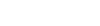The current in the ether caused the dielectric displacement and the spin at R is: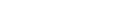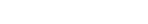When the rotation speed reaches c the passing of charge between particle –Qe and the surrounding ether will end. The inert quality of ether prevents the dielectric displacement to be dragged any further. Charge –Qe is now transferred from the negative point-volume to the surface of a bulb with radius Re. In the rotating profile R<Re the speed of rotation is: Vr=cR/ReIntegration of dIr over 2π radials and dr from 0 to Re gives: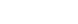The dielectric displacement takes place on both sides of the turning “disc”, so the total surface current Ic of the spin is: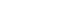The energy of current dIr is: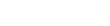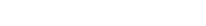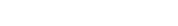The surface current of the spinning disc induces on both sides a current in the ether: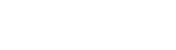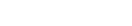The energy Wm presents the magnetic spin energy of the electron. The expansion of charge Qe over space comes to an end when the turnover speed becomes c at Re. The charge Qe is transferred from the negative point-volume to the “surface” of a bulb with a radius Re; the potential energy is given :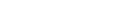The total energy of the electron We is the sum of the dynamic magnetic energy Wm and the electrostatic energy Wp; We=Wm+Wp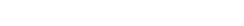With the equivalence of mass and energy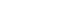the radius Re of the electron is calculated atmeter; exact the Compton Radius.

Next chapter: The equivalence of magnetic and kinetic energyAutomated page speed optimizations for fast site performance